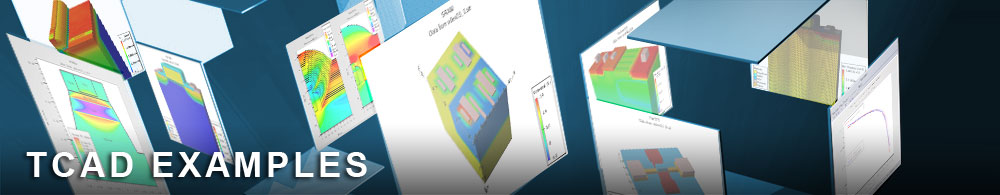Coupled Mode Space NEGF approach to Double Gate FET

## quantumex12.in : Coupled Mode Space NEGF approach to Double Gate FET

Requires: S-Pisces/Quantum
Minimum Versions: Atlas 5.28.1.R

This example demonstrates:

• Use of NEGF_MS model
• Quantum confinement, ballistic transport

This is an example of modeling the ballistic I-V characteristics of a nanoscale double gate transistor using the Non Equilibrium Green's function approach. Atlas solves a 1D Schrodinger equation in order to find eigenvalues and eigenfunctions at each slice of the device. A quantum transport equation (NEGF) is then solved. Due to a varying cross-section of the transistors, Atlas will automatically account for coupling between subbands, which is called the coupled modespace approach.

First, a rectangular mesh is created. A spacing in the transport direction should be fine enough, so that a half-bandwidth of discretized effective mass Hamiltonian is larger than applied bias. Here we consider a simple double gate transistor with flared up source and drain extensions. Device length is 35 nm, channel thickness is 1 nm, and gate oxide is 1 nm gate length is 10nm. Source and drain extension thickness is 5nm. Only two carrier injecting contacts (source and drain) are supported in Atlas. There can be any number of gates.

Due to the ballistic nature of transport, there is no voltage drop near the source and drain contacts. Moreover, the potential in the source and drain has to be allowed to float because the number of electrons reflected backward and thus total carrier concentration in contacts can change with bias.

For these reasons we use REFLECT on the CONTACT statement to apply von Neumann boundary conditions for potential. Note that Fermi levels in contacts are fixed.

SCHRODINGER and/or P.SCHRODINGER parameters on the MODELS statement will tell Atlas that this is a quantum solution. A Schrodinger equation will be solved in each slice of the device for electrons and/or holes. NEGF_MS parameter specifies that we want to compute transport using Non Equilibrium Green's Function (NEGF) approach in the mode (subband) space. If the cross-section is not constant, Atlas will take into account coupling between subbands.

The coupling is especially strong near the wide-narrow region interface. A parameter NEGF_CMS instead of NEGF_MS will enforce the coupled mode space approach. A parameter NEGF_UMS instead of NEGF_MS will enforce uncoupled mode space approach. Parameter EIGEN can be omitted on the MODELS statement. Atlas will automatically decide on the optimum number of subbands required. This number may change with bias. Setting the EIGEN parameter will fix the number of subbands, which may either cause a loss of precision (if it is too small), or increase the computation time (if it is too large).

Note that the number of subbands taken into account may vary along the device. Parameters OX.SCHRO and OX.MARGIN control wavefunction penetration into oxide. This ensures that electron density is smooth at the semiconductor-oxide interface. Here, a parameter NUM.DIRECT is set to 1, which means that only one isotropic mass is taken into account.

When all NUM.DIRECT=3, all three pairs of valleys (in the case of Si) are treated independently. Note that while primed subbands have the same eigen energy due to the same effective mass in the confinement direction, their transport effective mass is different. Therefore, transmission and current due to primed subbands are different.

A predictor-corrector scheme for Poisson convergence is used, where after each quantum solution (corrector), a quasi Fermi level is extracted and several iterations with classical electron density (predictor) are performed. NPRED.NEGF=7 (default) sets the number of predictor iterations to 7 QCRIT.NEGF=3e-3 (default) sets the potential convergence criterium in the NEGF case to 3 mV ESIZE.NEGF=2001 (default) sets the number of energy grid points to 2001. A nonuniform energy grid is constructed. A larger number may improve precision in the electron density calculation and improve convergence, but increase the computation time. Parameter CARRIERS on the METHOD statement is set to zero which means that only a Poisson equation is solved self-consistently with NEGF equations.

In the structure file, quantities of interest are potential, carrier density, current density profiles, bound state energies and wavefunctions. A user can also probe various energy dependent quantities, which are specific to NEGF method, and store them in a file at each bias.

Set NEGF.LOG parameter on SOLVE or SAVE statement to save the probes. ESIZEOUT.NEGF=500 (default) sets the number of energy grid points to 500 in the output .log file if the PROBE statements are present (see below). A uniform energy grid is used. The Parameter FILENAME can be specified only once. In this case a file TransvsE\$.log will be created at each bias point, where \$ is the number of the bias point. Comment lines in the file give the values of terminal bias. All probes are plotted as functions of Energy. A uniform energy grid is used.

The size of the grid is regulated in the OUTPUT statement (see above). TRANSMISSION is the number of modes that can pass through the device at a particular energy. One can also separate contributions due to each pair of valleys using the parameter BAND. DOSVSE is the local density of states. It can be characterized by the parameters BAND, STATE and location X. DENSVSE is the local carrier density versus Energy, and integrating DENSVSE gives the total carrier concentration at this location. CURDVSE is the local current density versus energy, and integrating CURDSVE gives the total current density at this location. In the ballistic case, i.e. when no inelastic phonons are present, this quantity should not change with location. It can be characterized by the parameters BAND, STATE and location X. If the parameters BAND or STATE are omitted, a summation is performed over the band or state indices.

Warning: Poor convergence usually results when there is no injection barrier.

To load and run this example, select the Load button in DeckBuild > Examples. This will copy the input file and any support files to your current working directory. Select the Run button in DeckBuild to execute the example.